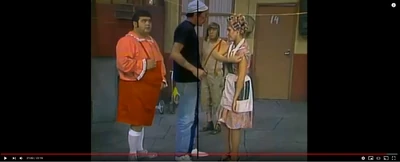26,593 Pages

## All Credits goes to Stefano4444 for the calcDon Ramon = 748 pixels (Black Line) = 1.78 m

Don Ramon's Trajectory (1) = 656 pixels (red Line) = 1.78*(656/748) = 1.56106952 m

Don Ramon's Trajectory (2) = 523 pixels (red Line) = 1.78*(523/748) = 1.24457219 m

Don Ramon's Trajectory (3) = 508 pixels (red Line) = 1.78*(508/748) = 1.20887701 m

Distance = 1.56106952+1.24457219+1.20887701 = 4.01451872 m

Kick start and end from 21:45:48 to 21:46:24

Time = (46.24)-(45.48) = 0.76 seconds

Speed = 4.01451872/0.76 = 5.28226147 m/s

KE = 1/2*(70)*(5.28226147)^2 = 976.580018 Joules or Street level

Community content is available under CC-BY-SA unless otherwise noted.